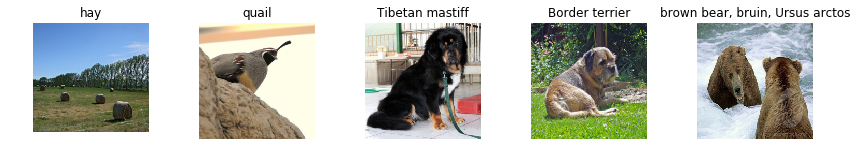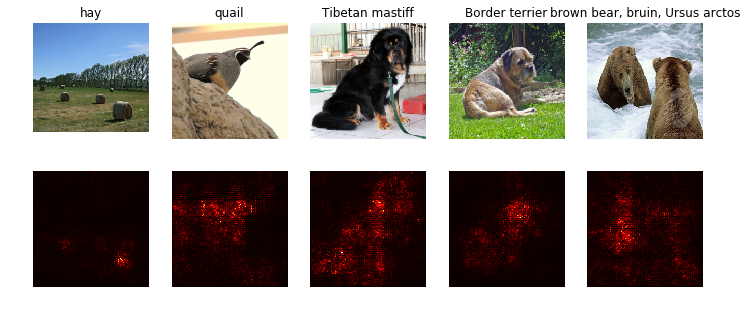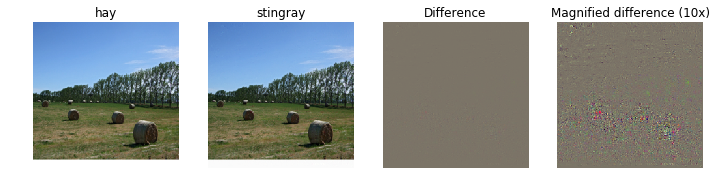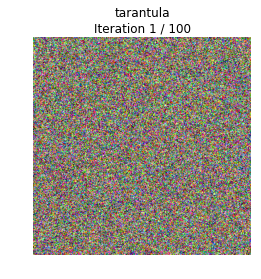# Network Visualization (PyTorch)¶

In this notebook we will explore the use of image gradients for generating new images.

When training a model, we define a loss function which measures our current unhappiness with the model's performance; we then use backpropagation to compute the gradient of the loss with respect to the model parameters, and perform gradient descent on the model parameters to minimize the loss.

Here we will do something slightly different. We will start from a convolutional neural network model which has been pretrained to perform image classification on the ImageNet dataset. We will use this model to define a loss function which quantifies our current unhappiness with our image, then use backpropagation to compute the gradient of this loss with respect to the pixels of the image. We will then keep the model fixed, and perform gradient descent on the image to synthesize a new image which minimizes the loss.

In this notebook we will explore three techniques for image generation:

1. Saliency Maps: Saliency maps are a quick way to tell which part of the image influenced the classification decision made by the network.
2. Fooling Images: We can perturb an input image so that it appears the same to humans, but will be misclassified by the pretrained network.
3. Class Visualization: We can synthesize an image to maximize the classification score of a particular class; this can give us some sense of what the network is looking for when it classifies images of that class.

This notebook uses PyTorch; we have provided another notebook which explores the same concepts in TensorFlow. You only need to complete one of these two notebooks.

In :
import torch
import torchvision
import torchvision.transforms as T
import random

import numpy as np
from scipy.ndimage.filters import gaussian_filter1d
import matplotlib.pyplot as plt
from cs231n.image_utils import SQUEEZENET_MEAN, SQUEEZENET_STD
from PIL import Image

%matplotlib inline
plt.rcParams['figure.figsize'] = (10.0, 8.0) # set default size of plots
plt.rcParams['image.interpolation'] = 'nearest'
plt.rcParams['image.cmap'] = 'gray'


### Helper Functions¶

Our pretrained model was trained on images that had been preprocessed by subtracting the per-color mean and dividing by the per-color standard deviation. We define a few helper functions for performing and undoing this preprocessing. You don't need to do anything in this cell.

In :
def preprocess(img, size=224):
transform = T.Compose([
T.Scale(size),
T.ToTensor(),
T.Normalize(mean=SQUEEZENET_MEAN.tolist(),
std=SQUEEZENET_STD.tolist()),
T.Lambda(lambda x: x[None]),
])
return transform(img)

def deprocess(img, should_rescale=True):
transform = T.Compose([
T.Lambda(lambda x: x),
T.Normalize(mean=[0, 0, 0], std=(1.0 / SQUEEZENET_STD).tolist()),
T.Normalize(mean=(-SQUEEZENET_MEAN).tolist(), std=[1, 1, 1]),
T.Lambda(rescale) if should_rescale else T.Lambda(lambda x: x),
T.ToPILImage(),
])
return transform(img)

def rescale(x):
low, high = x.min(), x.max()
x_rescaled = (x - low) / (high - low)
return x_rescaled

def blur_image(X, sigma=1):
X_np = X.cpu().clone().numpy()
X_np = gaussian_filter1d(X_np, sigma, axis=2)
X_np = gaussian_filter1d(X_np, sigma, axis=3)
X.copy_(torch.Tensor(X_np).type_as(X))
return X


# Pretrained Model¶

For all of our image generation experiments, we will start with a convolutional neural network which was pretrained to perform image classification on ImageNet. We can use any model here, but for the purposes of this assignment we will use SqueezeNet , which achieves accuracies comparable to AlexNet but with a significantly reduced parameter count and computational complexity.

Using SqueezeNet rather than AlexNet or VGG or ResNet means that we can easily perform all image generation experiments on CPU.

 Iandola et al, "SqueezeNet: AlexNet-level accuracy with 50x fewer parameters and < 0.5MB model size", arXiv 2016

In :
# Download and load the pretrained SqueezeNet model.
model = torchvision.models.squeezenet1_1(pretrained=True)

# We don't want to train the model, so tell PyTorch not to compute gradients
# with respect to model parameters.
for param in model.parameters():


We have provided a few example images from the validation set of the ImageNet ILSVRC 2012 Classification dataset. To download these images, change to cs231n/datasets/ and run get_imagenet_val.sh.

Since they come from the validation set, our pretrained model did not see these images during training.

Run the following cell to visualize some of these images, along with their ground-truth labels.

In :
from cs231n.data_utils import load_imagenet_val

plt.figure(figsize=(12, 6))
for i in range(5):
plt.subplot(1, 5, i + 1)
plt.imshow(X[i])
plt.title(class_names[y[i]])
plt.axis('off')
plt.gcf().tight_layout()# Saliency Maps¶

Using this pretrained model, we will compute class saliency maps as described in Section 3.1 of .

A saliency map tells us the degree to which each pixel in the image affects the classification score for that image. To compute it, we compute the gradient of the unnormalized score corresponding to the correct class (which is a scalar) with respect to the pixels of the image. If the image has shape (3, H, W) then this gradient will also have shape (3, H, W); for each pixel in the image, this gradient tells us the amount by which the classification score will change if the pixel changes by a small amount. To compute the saliency map, we take the absolute value of this gradient, then take the maximum value over the 3 input channels; the final saliency map thus has shape (H, W) and all entries are nonnegative.

 Karen Simonyan, Andrea Vedaldi, and Andrew Zisserman. "Deep Inside Convolutional Networks: Visualising Image Classification Models and Saliency Maps", ICLR Workshop 2014.

### Hint: PyTorch gather method¶

Recall in Assignment 1 you needed to select one element from each row of a matrix; if s is an numpy array of shape (N, C) and y is a numpy array of shape (N,) containing integers 0 <= y[i] < C, then s[np.arange(N), y] is a numpy array of shape (N,) which selects one element from each element in s using the indices in y.

In PyTorch you can perform the same operation using the gather() method. If s is a PyTorch Tensor or Variable of shape (N, C) and y is a PyTorch Tensor or Variable of shape (N,) containing longs in the range 0 <= y[i] < C, then

s.gather(1, y.view(-1, 1)).squeeze()

will be a PyTorch Tensor (or Variable) of shape (N,) containing one entry from each row of s, selected according to the indices in y.

run the following cell to see an example.

You can also read the documentation for the gather method and the squeeze method.

In :
# Example of using gather to select one entry from each row in PyTorch
def gather_example():
N, C = 4, 5
s = torch.randn(N, C)
y = torch.LongTensor([1, 2, 1, 3])
print(s)
print(y)
print(s.gather(1, y.view(-1, 1)).squeeze())
gather_example()

-0.9695  0.3291 -0.4649 -0.7073  1.7230
-0.9341  1.8693 -0.8987  0.0674  0.9908
0.5223 -1.0662 -0.6448  0.9887 -0.1528
1.2723  0.6175  0.2850 -0.0211 -2.0851
[torch.FloatTensor of size 4x5]

1
2
1
3
[torch.LongTensor of size 4]

0.3291
-0.8987
-1.0662
-0.0211
[torch.FloatTensor of size 4]


In :
def compute_saliency_maps(X, y, model):
"""
Compute a class saliency map using the model for images X and labels y.

Input:
- X: Input images; Tensor of shape (N, 3, H, W)
- y: Labels for X; LongTensor of shape (N,)
- model: A pretrained CNN that will be used to compute the saliency map.

Returns:
- saliency: A Tensor of shape (N, H, W) giving the saliency maps for the input
images.
"""
# Make sure the model is in "test" mode
model.eval()

# Wrap the input tensors in Variables
y_var = Variable(y)
saliency = None
##############################################################################
# TODO: Implement this function. Perform a forward and backward pass through #
# the model to compute the gradient of the correct class score with respect  #
# to each input image. You first want to compute the loss over the correct   #
# scores, and then compute the gradients with a backward pass.               #
##############################################################################
scores = model(X_var)
scores = scores.gather(1, y_var.view(-1, 1)).squeeze()
loss = -torch.sum(torch.log(scores))
loss.backward()
saliency = saliency.abs()
saliency, idx = saliency.max(dim=1)
##############################################################################
#                             END OF YOUR CODE                               #
##############################################################################
return saliency.squeeze()


Once you have completed the implementation in the cell above, run the following to visualize some class saliency maps on our example images from the ImageNet validation set:

In :
def show_saliency_maps(X, y):
# Convert X and y from numpy arrays to Torch Tensors
X_tensor = torch.cat([preprocess(Image.fromarray(x)) for x in X], dim=0)
y_tensor = torch.LongTensor(y)

# Compute saliency maps for images in X
saliency = compute_saliency_maps(X_tensor, y_tensor, model)

# Convert the saliency map from Torch Tensor to numpy array and show images
# and saliency maps together.
saliency = saliency.numpy()
N = X.shape
for i in range(N):
plt.subplot(2, N, i + 1)
plt.imshow(X[i])
plt.axis('off')
plt.title(class_names[y[i]])
plt.subplot(2, N, N + i + 1)
plt.imshow(saliency[i], cmap=plt.cm.hot)
plt.axis('off')
plt.gcf().set_size_inches(12, 5)
plt.show()

show_saliency_maps(X, y)# Fooling Images¶

We can also use image gradients to generate "fooling images" as discussed in . Given an image and a target class, we can perform gradient ascent over the image to maximize the target class, stopping when the network classifies the image as the target class. Implement the following function to generate fooling images.

 Szegedy et al, "Intriguing properties of neural networks", ICLR 2014

In :
def make_fooling_image(X, target_y, model):
"""
Generate a fooling image that is close to X, but that the model classifies
as target_y.

Inputs:
- X: Input image; Tensor of shape (1, 3, 224, 224)
- target_y: An integer in the range [0, 1000)
- model: A pretrained CNN

Returns:
- X_fooling: An image that is close to X, but that is classifed as target_y
by the model.
"""
# Initialize our fooling image to the input image, and wrap it in a Variable.
X_fooling = X.clone()

learning_rate = 1
##############################################################################
# TODO: Generate a fooling image X_fooling that the model will classify as   #
# the class target_y. You should perform gradient ascent on the score of the #
# target class, stopping when the model is fooled.                           #
# When computing an update step, first normalize the gradient:               #
#   dX = learning_rate * g / ||g||_2                                         #
#                                                                            #
# You should write a training loop.                                          #
#                                                                            #
# HINT: For most examples, you should be able to generate a fooling image    #
# in fewer than 100 iterations of gradient ascent.                           #
# You can print your progress over iterations to check your algorithm.       #
##############################################################################
while True:
scores = model(X_fooling_var)
pred_idx = scores.data.max(dim=1)
if pred_idx!=target_y:
scores[:,target_y].backward()
X_fooling += dX
else:
break
##############################################################################
#                             END OF YOUR CODE                               #
##############################################################################
return X_fooling


Run the following cell to generate a fooling image:

In :
idx = 0
target_y = 6

X_tensor = torch.cat([preprocess(Image.fromarray(x)) for x in X], dim=0)
X_fooling = make_fooling_image(X_tensor[idx:idx+1], target_y, model)

scores = model(Variable(X_fooling))
assert target_y == scores.data.max(1), 'The model is not fooled!'


After generating a fooling image, run the following cell to visualize the original image, the fooling image, as well as the difference between them.

In :
X_fooling_np = deprocess(X_fooling.clone())
X_fooling_np = np.asarray(X_fooling_np).astype(np.uint8)

plt.subplot(1, 4, 1)
plt.imshow(X[idx])
plt.title(class_names[y[idx]])
plt.axis('off')

plt.subplot(1, 4, 2)
plt.imshow(X_fooling_np)
plt.title(class_names[target_y])
plt.axis('off')

plt.subplot(1, 4, 3)
X_pre = preprocess(Image.fromarray(X[idx]))
diff = np.asarray(deprocess(X_fooling - X_pre, should_rescale=False))
plt.imshow(diff)
plt.title('Difference')
plt.axis('off')

plt.subplot(1, 4, 4)
diff = np.asarray(deprocess(10 * (X_fooling - X_pre), should_rescale=False))
plt.imshow(diff)
plt.title('Magnified difference (10x)')
plt.axis('off')

plt.gcf().set_size_inches(12, 5)
plt.show()# Class visualization¶

By starting with a random noise image and performing gradient ascent on a target class, we can generate an image that the network will recognize as the target class. This idea was first presented in ;  extended this idea by suggesting several regularization techniques that can improve the quality of the generated image.

Concretely, let $I$ be an image and let $y$ be a target class. Let $s_y(I)$ be the score that a convolutional network assigns to the image $I$ for class $y$; note that these are raw unnormalized scores, not class probabilities. We wish to generate an image $I^*$ that achieves a high score for the class $y$ by solving the problem

$$I^* = \arg\max_I s_y(I) - R(I)$$

where $R$ is a (possibly implicit) regularizer (note the sign of $R(I)$ in the argmax: we want to minimize this regularization term). We can solve this optimization problem using gradient ascent, computing gradients with respect to the generated image. We will use (explicit) L2 regularization of the form

$$R(I) = \lambda \|I\|_2^2$$

and implicit regularization as suggested by  by periodically blurring the generated image. We can solve this problem using gradient ascent on the generated image.

In the cell below, complete the implementation of the create_class_visualization function.

 Karen Simonyan, Andrea Vedaldi, and Andrew Zisserman. "Deep Inside Convolutional Networks: Visualising Image Classification Models and Saliency Maps", ICLR Workshop 2014.

 Yosinski et al, "Understanding Neural Networks Through Deep Visualization", ICML 2015 Deep Learning Workshop

In :
def jitter(X, ox, oy):
"""
Helper function to randomly jitter an image.

Inputs
- X: PyTorch Tensor of shape (N, C, H, W)
- ox, oy: Integers giving number of pixels to jitter along W and H axes

Returns: A new PyTorch Tensor of shape (N, C, H, W)
"""
if ox != 0:
left = X[:, :, :, :-ox]
right = X[:, :, :, -ox:]
X = torch.cat([right, left], dim=3)
if oy != 0:
top = X[:, :, :-oy]
bottom = X[:, :, -oy:]
X = torch.cat([bottom, top], dim=2)
return X

In :
def create_class_visualization(target_y, model, dtype, **kwargs):
"""
Generate an image to maximize the score of target_y under a pretrained model.

Inputs:
- target_y: Integer in the range [0, 1000) giving the index of the class
- model: A pretrained CNN that will be used to generate the image
- dtype: Torch datatype to use for computations

Keyword arguments:
- l2_reg: Strength of L2 regularization on the image
- learning_rate: How big of a step to take
- num_iterations: How many iterations to use
- blur_every: How often to blur the image as an implicit regularizer
- max_jitter: How much to gjitter the image as an implicit regularizer
- show_every: How often to show the intermediate result
"""
model.type(dtype)
l2_reg = kwargs.pop('l2_reg', 1e-3)
learning_rate = kwargs.pop('learning_rate', 25)
num_iterations = kwargs.pop('num_iterations', 100)
blur_every = kwargs.pop('blur_every', 10)
max_jitter = kwargs.pop('max_jitter', 16)
show_every = kwargs.pop('show_every', 25)

# Randomly initialize the image as a PyTorch Tensor, and also wrap it in
# a PyTorch Variable.
img = torch.randn(1, 3, 224, 224).mul_(1.0).type(dtype)

for t in range(num_iterations):
# Randomly jitter the image a bit; this gives slightly nicer results
ox, oy = random.randint(0, max_jitter), random.randint(0, max_jitter)
img.copy_(jitter(img, ox, oy))

########################################################################
# TODO: Use the model to compute the gradient of the score for the     #
# class target_y with respect to the pixels of the image, and make a   #
# gradient step on the image using the learning rate. Don't forget the #
# L2 regularization term!                                              #
# Be very careful about the signs of elements in your code.            #
########################################################################
scores = model(img_var)
scores[:,target_y].backward()
########################################################################
#                             END OF YOUR CODE                         #
########################################################################

# Undo the random jitter
img.copy_(jitter(img, -ox, -oy))

# As regularizer, clamp and periodically blur the image
for c in range(3):
lo = float(-SQUEEZENET_MEAN[c] / SQUEEZENET_STD[c])
hi = float((1.0 - SQUEEZENET_MEAN[c]) / SQUEEZENET_STD[c])
img[:, c].clamp_(min=lo, max=hi)
if t % blur_every == 0:
blur_image(img, sigma=0.5)

# Periodically show the image
if t == 0 or (t + 1) % show_every == 0 or t == num_iterations - 1:
plt.imshow(deprocess(img.clone().cpu()))
class_name = class_names[target_y]
plt.title('%s\nIteration %d / %d' % (class_name, t + 1, num_iterations))
plt.gcf().set_size_inches(4, 4)
plt.axis('off')
plt.show()

return deprocess(img.cpu())


Once you have completed the implementation in the cell above, run the following cell to generate an image of a Tarantula:

In :
dtype = torch.FloatTensor
# dtype = torch.cuda.FloatTensor # Uncomment this to use GPU
model.type(dtype)

target_y = 76 # Tarantula
# target_y = 78 # Tick
# target_y = 187 # Yorkshire Terrier
# target_y = 683 # Oboe
# target_y = 366 # Gorilla
# target_y = 604 # Hourglass
out = create_class_visualization(target_y, model, dtype)